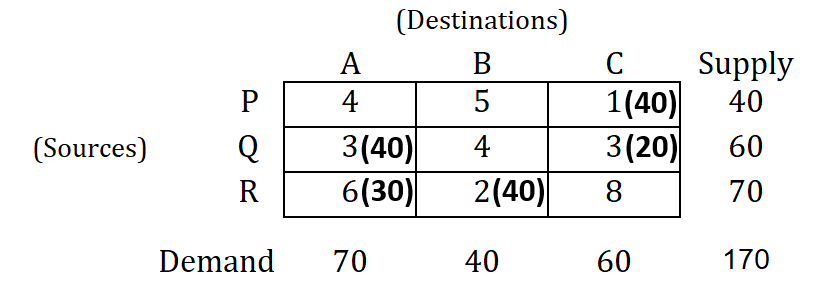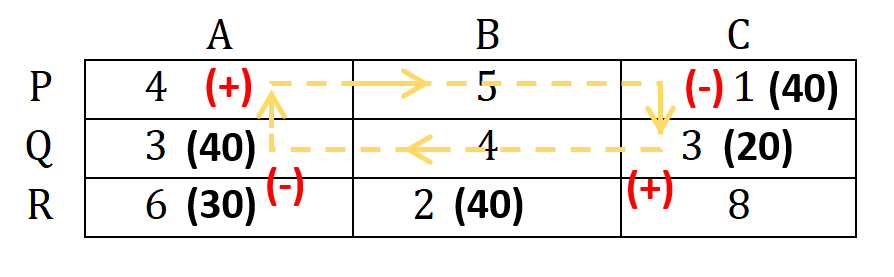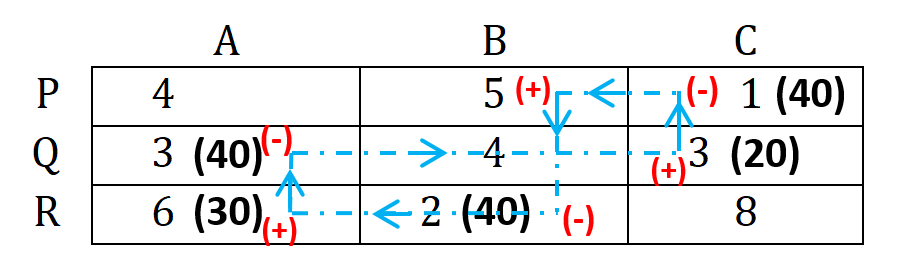# Stepping Stone | Transportation Problem | Transportation Model

Comments

As we discussed in MODI method that, to understand these methods which will be used to get optimum solution to transportation problem, we need to get knowledge of optimality test first.

Jump to Stepping Stone Method or Modified Distribution Method (MODI) for optimizing solution to transportation problem.

## Optimality Test :

→ Optimality test is carried to check whether the basic feasible solution (BFS) obtained by any of different methods ( Vogel's Approximation Method (VAM), Least Cost Method or North West Corner Method | Method to Solve Transportation Problem | Transportation Model ) is optimum or not!

→ This test is done only after fulfilling two requirements as follows:

a) Total number of non-negative allocation is (m+n-1).

b) These allocations are in independent cells.

where,
m = no. of rows &
n = no. of columns

→ There are two different methods used for checking whether the solution to transportation problem is optimal or not.

## Stepping Stone Method:

→ In basic feasible solution (BFS), there will be unallocated cells. In this method, for checking the optimality of the solution obtained, we arbitrarily select any of the unoccupied cells and then we allocate units to that unit, and subsequently we deduct from other cells in the matrix, such that supply and demand are not altered.

→ If net change in cost comes out negative, then we conclude that the new allocated cell can improve the BFS and the present solution has opportunity for improvement.

→ If net change in cost comes out positive for that allocated cell , then the present solution is optimal.

→ In this method, this procedure is carried out for all vacant cells. If any of unallocated cell gives net change in cost as negative, then from that cell we can improve the present solution.

Let us now understand how one can apply Stepping Stone Method. We will understand it using a numerical as follows:

## Applying Stepping Stone Method - Numerical

### Question : Check optimality of the basic feasible solution given below by using Stepping Stone method.Fig-1: Question

### Solution :

Note that all the explanation is provided in “CYAN” colour. You have to write in examination the only thing which are given in this regular colour under each steps (if any), else you can directly solve matrix of the problem as explained here.

→ Here in the given numerical the unoccupied/unallocated cells are: PA, PB, QB & RC.Fig-2: Creating PA → PC → QC → QA loop for unallocated cell PA

→ Draw the closed loop (as shown above) from cell PA (unallocated cell) and complete the loop by using allocated cells.

→ Closed loop as following will be formed :

PA → PC → QC → QA

→ Mark (+) & (-) sign alternatively at each corner, starting from the original unallocated cell as shown above.

→ Find the net change in the cost:
For PA cell; net change in cost will be;

$+4-1+3-3 = 3$

→ Similarly, repeat the procedure for all unallocated cells.Rules to create closed loops:

• Start a loop from unallocated cell and then use allocated cells to complete the loop.
• Only horizontal and vertical movements are allowed and for once at a time. Diagonal movements are not allowed.
• Only two cells should be used in a specific row or column while creating a loop.

For explanation purpose we are showing extra step here, illustrating allocation of cell PB.

You can show all allocations in the same matrix in the examination, but add the table as shown below.
Use different lines for different loops, so that you and supervisor both can easily distinguish between loops.

Tips for drawing lines for loops :
→ Use ball-point pen and pencil to get two different lines.
→ If you have black pen then, draw one loop with black colour.
→ Upon limitation to colour pens and pencil, you can use different line patterns as straight line, dashed lines, curvy lines, centre lines, etc.Fig-3: Creating PB → RB → RA → QA → QC → PC loop for unallocated cell PB

Unallocated cells Closed loop Net cost change
PA PA → PC → QC → QA $+4-1+3-3=3$
PB PB → RB → RA → QA → QC → PC $5-2+6-3+3-1=8$
QB QB → QA → RA → RB $4-3+6-2=5$
RC RC → QC → QA → RA $8-3+3-6=2$

### Note that :

In Stepping Stone method, if the net cost change are/is:

(i) $\ge 0$ → then the current basic feasible solution (BFS) is optimal and stop the procedure.

(ii) $< 0$ → then the current basic feasible solution (BFS) is not optimal and further improvement is possible.

→ As here all the net cost change are positive (+) or $\ge 0$, the present solution is optimal.

## Limitations of Stepping Stone Method:

→ In Stepping Stone Method method there are 'm' rows and 'n' columns, so the total number of unoccupied cell will be equal to $m⋅n−(m+n−1)$.

→ Thus, total number of loops increases, which increases calculation and it will be more time consuming.

Check optimality of same problem using Modified Distribution Method (MODI).

### Comments:

All comments that you add will await moderation. We'll publish all comments that are topic related, and adhere to our Code of Conduct.

Want to tell us something privately? Contact Us